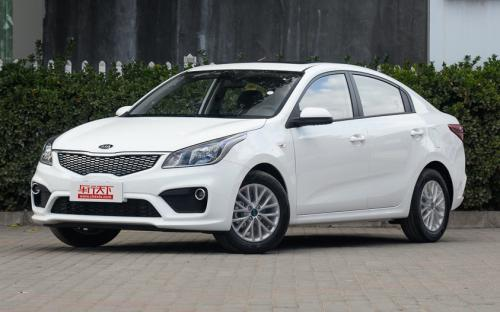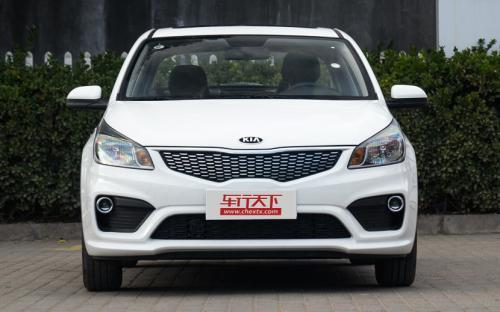### 东风悦达起亚 起亚K20 种颜色可选2017款最低售价：7.29 万元起

4400(mm)1740(mm)1460(mm)##### 配置亮点：
• 胎压监测装置

• ISOFIX儿童座椅接口

• 车身稳定控制(ESC/ESP/DSC等)

• 电动天窗

• 定速巡航

• 后倒车雷达

• 真皮座椅

• GPS导航系统

• 氙气大灯

• 后视镜加热

• 提交
2017款 三厢 1.4L AT GLS (130张)

东风悦达起亚 起亚K2 绕车实拍• 东风悦达起亚 起亚K2 在售车型

排量 车型 厂商指导价 本地最低报价 购车工具
1.4L
三厢 1.4L MT GL 6挡手动
7.29万
三厢 1.4L MT GLS 6挡手动
7.79万
三厢 1.4L AT GLS 6挡手自一体
8.89万
三厢 1.4L MT DLX 6挡手动
8.59万
10.39万
1.6L
三厢 1.4L MT GL 6挡手动
7.29万
三厢 1.4L MT GLS 6挡手动
7.79万
三厢 1.4L AT GLS 6挡手自一体
8.89万
三厢 1.4L MT DLX 6挡手动
8.59万
10.39万

东风悦达起亚 起亚K2 经销商

查看更多 >>

### 东风悦达起亚 起亚K2 动力加速

起亚K2 0-100公里加速时间分布在 0.0-秒 属于 超跑级

动力级别 加速时间 车型

东风悦达起亚 起亚K2 视频

东风悦达起亚 起亚K2 新闻资讯

# 三款节油中级家轿推介

新闻中心 超过4050次关注

选择8万元以下车型的用户，是对用车成本最敏感的群体，因此酷炫的高科技和动力充沛的表现固然是加分项，但是实用性和经济性才是普通汽车消费人群最根本的追求。为此...

# 外观内饰小改 东风悦达起亚新款K2实车

国产新车 超过5330次关注

日前，我们拍摄到东风悦达起亚新款K2的实车图片，相比老款，其针对造型设计进行了改进。据悉，新款K2将会在11月20日开幕的广州车展首发。

# 11月首发 东风悦达起亚新款K2官图曝光

国产新车 超过5736次关注

日前，我们获得了东风悦达起亚新款K2的图片。据悉，这款车将会在11月的广州车展首发。

# 起亚新款K2内饰谍照曝光 或年底推出

新闻 超过5738次关注

日前，汽车之家网友向我们提供了一组东风悦达起亚新款K2的内饰谍照。据此前报道，新款车型预计在2014年底推出。

# 花样年华 试驾东风悦达起亚K2两厢

车系文章 超过4093次关注

东风悦达起亚K2两厢优点：轴距优势保证了足够的车内空间缺点：4挡自动变速器的反应有点慢

# 起亚“小K2”推双燃料版 百公里油耗5.6L

车系文章 超过2160次关注

小型化和节能环保已经成为汽车发展的趋势，起亚将在这两方面强化布局。网通社近日获悉，定位低于K2的微型车-Picanto不仅使起亚正式进军此细分市场，其还将在即将开...

# 意料之中的结果 车展体验两厢版起亚K2

车系文章 超过4215次关注

2011年3月，第三代起亚Rio（两厢车）在日内瓦车展正式上市。同年7月，东风悦达起亚也推出了定位高于国内锐欧RIO的全新小型车，并命名为K2（三厢）。应该说国内的这...

# 售7.29-9.99万元 起亚K2两厢版正式上市

车系文章 超过2984次关注

2月20日，东风悦达起亚官方宣布，K2两厢版正式上市。此次上市的新车共有2个排量4款车型，两厢相关车款的售价和三厢相同，两厢版4款车型的售价为7.29-9.99万元。

# 东风悦达起亚将推出K2十周年特别版车型

车系文章 超过2459次关注

继东风悦达起亚推出K5十周年特别版车型之后，日前，我们从官方获悉，东风悦达起亚还将推出K2的十周年特别版车型，其中K2三厢版被命名为K2十周年纪念版，而两厢版车...

# 起亚K2店内有现车销售 购车优惠4000元

车系文章 超过3217次关注

近日，编辑从河南普泽汽车销售服务有限公司了解到，目前店内起亚K2现车销售，购车可优惠4000元，感兴趣的朋友不妨到店了解。

猜你喜欢

﻿
• 快速找车
• 选择品牌
• 选择品牌
• A  奥迪
• A  阿斯顿·马丁
• A  阿尔法·罗密欧
• B  宝沃
• B  布加迪
• B  巴博斯
• B  保时捷
• B  宾利
• B  奔驰
• B  宝马
• B  本田
• B  别克
• B  标致
• B  比亚迪
• B  宝骏
• B  北汽制造
• B  北汽新能源
• B  北汽幻速
• B  北汽威旺
• B  北京汽车
• B  奔腾
• B  北汽绅宝
• C  长安
• C  长安商用
• C  长城
• C  昌河
• D  大众
• D  道奇
• D  DS
• D  东南
• D  东风风神
• D  东风风行
• D  东风小康
• D  东风风度
• D  东风
• F  福特
• F  丰田
• F  菲亚特
• F  法拉利
• F  福田
• F  福迪
• F  福汽启腾
• G  观致
• G  广汽传祺
• G  广汽吉奥
• G  GMC
• H  红旗
• H  汉腾汽车
• H  哈弗
• H  哈飞
• H  海格
• H  海马
• H  华颂
• H  黄海
• H  华泰
• H  恒天
• J  吉利汽车
• J  捷豹
• J  Jeep
• J  江淮
• J  江铃
• J  金杯
• J  九龙
• J  金旅
• K  凯翼
• K  凯迪拉克
• K  克莱斯勒
• K  科尼塞克
• K  卡威
• K  开瑞
• L  路虎
• L  林肯
• L  劳斯莱斯
• L  兰博基尼
• L  雷克萨斯
• L  铃木
• L  雷诺
• L  理念
• L  力帆
• L  莲花汽车
• L  猎豹
• L  路特斯
• L  陆风
• M  马自达
• M  MG
• M  MINI
• M  玛莎拉蒂
• M  摩根
• M  迈凯轮
• N  纳智捷
• O  欧宝
• O  讴歌
• O  欧朗
• Q  奇瑞
• Q  起亚
• Q  启辰
• R  日产
• R  荣威
• R  瑞麒
• S  三菱
• S  斯威汽车
• S  萨博
• S  smart
• S  斯柯达
• S  斯巴鲁
• S  思铭
• S  双龙
• S  上汽大通
• S  双环
• T  特斯拉
• T  腾势
• W  沃尔沃
• W  五菱汽车
• W  五十铃
• W  威兹曼
• W  威麟
• X  现代
• X  雪佛兰
• X  雪铁龙
• X  西雅特
• Y  一汽
• Y  英菲尼迪
• Y  英致
• Y  依维柯
• Y  野马汽车
• Y  永源
• Z  众泰
• Z  中华
• Z  中兴
• Z  知豆
• 选择车系
• 选择车系
• 车型对比
• 选择品牌
• 选择品牌
• A  奥迪
• A  阿斯顿·马丁
• A  阿尔法·罗密欧
• B  宝沃
• B  布加迪
• B  巴博斯
• B  保时捷
• B  宾利
• B  奔驰
• B  宝马
• B  本田
• B  别克
• B  标致
• B  比亚迪
• B  宝骏
• B  北汽制造
• B  北汽新能源
• B  北汽幻速
• B  北汽威旺
• B  北京汽车
• B  奔腾
• B  北汽绅宝
• C  长安
• C  长安商用
• C  长城
• C  昌河
• D  大众
• D  道奇
• D  DS
• D  东南
• D  东风风神
• D  东风风行
• D  东风小康
• D  东风风度
• D  东风
• F  福特
• F  丰田
• F  菲亚特
• F  法拉利
• F  福田
• F  福迪
• F  福汽启腾
• G  观致
• G  广汽传祺
• G  广汽吉奥
• G  GMC
• H  红旗
• H  汉腾汽车
• H  哈弗
• H  哈飞
• H  海格
• H  海马
• H  华颂
• H  黄海
• H  华泰
• H  恒天
• J  吉利汽车
• J  捷豹
• J  Jeep
• J  江淮
• J  江铃
• J  金杯
• J  九龙
• J  金旅
• K  凯翼
• K  凯迪拉克
• K  克莱斯勒
• K  科尼塞克
• K  卡威
• K  开瑞
• L  路虎
• L  林肯
• L  劳斯莱斯
• L  兰博基尼
• L  雷克萨斯
• L  铃木
• L  雷诺
• L  理念
• L  力帆
• L  莲花汽车
• L  猎豹
• L  路特斯
• L  陆风
• M  马自达
• M  MG
• M  MINI
• M  玛莎拉蒂
• M  摩根
• M  迈凯轮
• N  纳智捷
• O  欧宝
• O  讴歌
• O  欧朗
• Q  奇瑞
• Q  起亚
• Q  启辰
• R  日产
• R  荣威
• R  瑞麒
• S  三菱
• S  斯威汽车
• S  萨博
• S  smart
• S  斯柯达
• S  斯巴鲁
• S  思铭
• S  双龙
• S  上汽大通
• S  双环
• T  特斯拉
• T  腾势
• W  沃尔沃
• W  五菱汽车
• W  五十铃
• W  威兹曼
• W  威麟
• X  现代
• X  雪佛兰
• X  雪铁龙
• X  西雅特
• Y  一汽
• Y  英菲尼迪
• Y  英致
• Y  依维柯
• Y  野马汽车
• Y  永源
• Z  众泰
• Z  中华
• Z  中兴
• Z  知豆
• 选择车系
• 选择车系
• 选择车型
• 选择车型
• 意见反馈